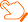### 近期论文查看导师最新文章 （温馨提示：请注意重名现象，建议点开原文通过作者单位确认）

Q. Liu, X. Le, and K. Li, “A distributed optimization algorithm based on multiagent network for economic dispatch with region partitioning,” IEEE Transactions on Cybernetics, 2019, in press. K. Li, Q. Liu, S. Yang, J. Cao, and G. Lu, “Cooperative optimization of dual multiagent system for optimal resource allocation,” IEEE Transactions on Systems, Man, and Cybernetics: Systems, 2019, in press. S. Yang, J. Wang, and Q. Liu, “Cooperative-competitive multiagent systems for distributed minimax optimization subject to bounded constraints,” IEEE Transactions on Automatic Control, vol. 64, no. 4, pp. 1358–1372, Apr. 2019. B. Xu, Q. Liu, and T. Huang, “A discrete-time projection neural network for sparse signal reconstruction with application to face recognition,” IEEE Transactions on Neural Networks and Learning Systems, vol. 30, no. 1, pp. 151–162, Jan. 2019. Q. Liu, S. Yang, and Y. Hong, “Constrained consensus algorithms with fixed step size for distributed convex optimization over multiagent networks,” IEEE Transactions on Automatic Control, vol. 62, no. 8, pp. 4259–4265, Aug. 2017. Q. Liu, S. Yang, and J. Wang, “A collective neurodynamic approach to distributed constrained optimization,” IEEE Transactions on Neural Networks and Learning Systems, vol. 28, no. 8, pp. 1747–1758, Aug. 2017. Q. Liu and J. Wang, “L1-minimization algorithms for sparse signal reconstruction based on a projection neural network,” IEEE Transactions on Neural Networks and Learning Systems, vol. 27, no. 3, pp. 698–707, Mar. 2016. Q. Liu and J. Wang, “A second-order multi-agent network for bound-constrained distributed optimization,” IEEE Transactions on Automatic Control, vol. 60, no. 12, pp. 3310–3315, Dec. 2015. Q. Liu and J. Wang, “A projection neural network for constrained quadratic minimax optimization,” IEEE Transactions on Neural Networks and Learning Systems, vol. 26, no. 11, pp. 2891–2900, Nov. 2015. Q. Liu, T. Huang, and J. Wang, “One-layer continuous- and discrete-time projection neural networks for solving variational inequalities and related optimization problems,” IEEE Transactions on Neural Networks and Learning Systems, vol. 25, no. 7, pp. 1308–1318, July 2014. Q. Liu and J. Wang, “A one-layer projection neural network for nonsmooth optimization subject to linear equalities and bound constraints,” IEEE Transactions on Neural Networks and Learning Systems, vol. 24, no. 5, pp. 812–824, May 2013. Q. Liu, C. Dang, and T. Huang, “A one-layer recurrent neural network for real-time portfolio optimization with probability criterion,” IEEE Transactions on Cybernetics, vol. 43, no. 1, pp. 14–23, Feb. 2013.

down
wechat